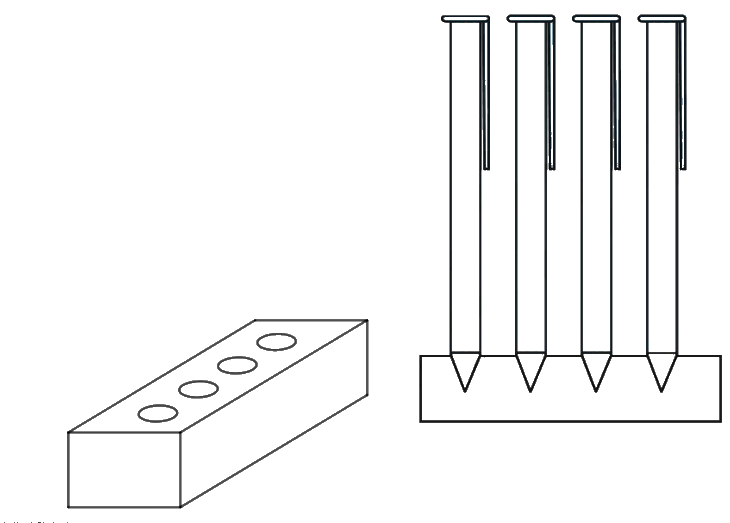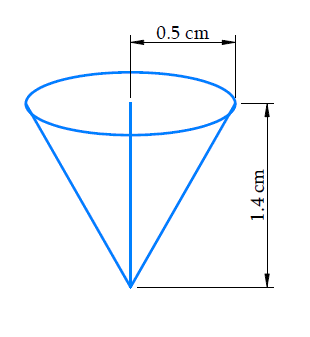In the verge of coronavirus pandemic, we are providing FREE access to our entire Online Curriculum to ensure Learning Doesn't STOP!

# Ex.13.2 Q4 Surface Areas and Volumes Solution - NCERT Maths Class 10

Go back to  'Ex.13.2'

## Question

A pen stand made of wood is in the shape of a cuboid with four conical depressions to hold pens. The dimensions of the cuboid are $$15 \,\rm{cm}$$ by $$10 \,\rm{cm}$$ by $$3.5 \,\rm{cm}$$. The radius of each of the depressions is $$0.5\,\rm{cm}$$ and the depth is $$1.4\,\rm{cm}$$.

Find the volume of wood in the entire stand (see Fig. 13.16).Fig. 13.16

Video Solution
Surface Areas And Volumes
Ex 13.2 | Question 4

## Text Solution

What is known?

A wooden pen stand is in the shape of a cuboid with four conical depressions.

The dimensions of the cuboid are $$15\rm\,cm\; \times 10\,cm \;\times\; 3.5\,cm$$

Radius of conical depressions is $$\,0.5\,\rm{cm}$$

Depth of conical depression is $$1.4\,\rm{cm}$$.

What is unknown?

Volume of wood in the entire pen stand.

Reasoning:

From the given figure it’s clear that the conical depressions do not contain wood. Since the dimensions of all $$4$$conical depressions are the same, they will have identical volumes too.

Volume of wood in the entire pen stand $$=$$ volume of the wooden cuboid $$- {\rm{ }}4 \times$$volume of the conical depression

We will find the volume of the solid by using formulae;

Volume of the cuboid$$= lbh$$

where $$l, b$$ and $$h$$ are the length, breadth and height of the cuboid respectively.

Volume of the cone\begin{align} = \frac{1}{3}\pi {r^2}{h_1}\end{align}

where $$r$$ and $$h_1$$ are the radius and height of the cone respectivelySteps:

Depth of each conical depression,$${h_1} = 1.4 \rm cm$$

Radius of each conical depression, $$r = 0.5 \rm cm$$

Dimensions of the cuboid are $$15 \rm cm \times 10cm \times 3.5cm$$

Volume of wood in the entire pen stand $$=$$ Volume of the wooden cuboid $$- 4 \;\times$$ Volume of each conical depression

\begin{align}&= lbh - 4 \times \frac{1}{3}\pi {r^2}{h_1}\\ &=\!\left(15 \rm cm \!\times\! 10cm \!\times\! 3.5 \rm cm\!\right) -\left( 4\!\times\! \frac{1}{3} \!\times\! \frac{{22}}{7}\!\times\! 0.5 \rm cm \!\times\! 0.5cm \!\times\! 1.4 \rm cm \right) \\&= 525 \rm c{m^3} - 1.47c{m^3}\\&= 523.53 \rm c{m^3}\end{align}

Learn from the best math teachers and top your exams

• Live one on one classroom and doubt clearing
• Practice worksheets in and after class for conceptual clarity
• Personalized curriculum to keep up with school